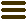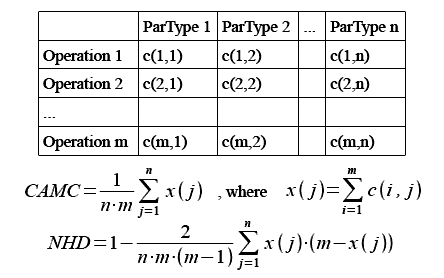The Software Design Metrics tool for the UMLCalculating Class Design Cohesion Metrics for UML

April 16, 2012, Jürgen Wüst. Category: Tips & Tricks

In an earlier post I have explained why SDMetrics does not feature any class cohesion metrics “out of the box”. In the following, I will show how you can use SDMetrics to calculate class cohesion metrics anyway. The metrics are:

• CAMC (Cohesion among methods in a class) by Bansiya et al, “A Class Cohesion Metric For Object-Oriented Designs”, JOOP 11(8): 47-52,1999
• NHD (Normalized Hamming Distance) by Counsell et al, “The interpretation and utility of three cohesion metrics for object-oriented design”, ACM TOSEM 15 (2): 123-149, 2006

I have picked these metrics because unlike most OO class cohesion metrics, they rely only on class interface information. Also, the metrics have received some attention in the literature. A good way of illustrating the definitions of these metrics is the “parameter occurrence matrix”, as shown below. Each row of the matrix represents one operation of a class. Each column represents an operation parameter type. The cell values c(i,j) are either 0 or 1 and indicate if the operation in row i has at least one parameter of the type in column j.Metric CAMC counts the percentage of “ones” in the matrix. Metric NHD compares all pairs of rows (operations), counting the number of columns for which the two rows have equal values, adds up this count over all row pairs, and normalizes the sum to give a maximum value of 1. If you do the math, you end up with the equation for NHD given above.

We’ll tackle CAMC first. Add the following SDMetricsML definitions to SDMetrics’ default metric definition file (the definitions work for both UML 1.x and UML 2.x):

<set name="OpParameterTypes" domain="operation">
<projection relation="context" target="parameter"
element="parametertype"/>
</set>

<set name="ClsParameterTypesMS" domain="class" multiset="true">
<projection relation="context" target="operation"
set="OpParameterTypes" />
</set>

The set “OpParameterTypes” collects the types of the parameters of one operation. This is a regular set; if an operation has multiple parameters with the same type, that type will still only be counted once. The set “ClsParameterTypesMS” takes the union of the parameter types over all operations of the class. This is a multiset; if a parameter type occurs in 15 operations, the cardinality of the parameter type in set “ClsParameterTypesMS” will also be 15.

With these two helper sets, we can define CAMC as follows:

<metric name="CAMC" domain="class" type="Cohesion">
<description>Cohesion among methods in the class.</description>
<compoundmetric fallback="0"
term="size(ClsParameterTypesMS)/(NumOps*flatsize(ClsParameterTypesMS))" />
</metric>

Metric “NumOps” is one of SDMetrics’ standard size metrics, and gives you the number of operations, m, in the above equation. The term “flatsize(ClsParameterTypesMS)” yields the number of parameter types, n.

For metric NHD, we add the following definitions to those for CAMC:

<set name="ClsParameterTypes" domain="class">
<projection relation="context" target="operation"
set="OpParameterTypes" />
</set>

<metric name="HD_raw" domain="class" internal="true">
<projection relset="ClsParameterTypes"
sum="(_self in _principal.ClsParameterTypesMS)*(_principal.NumOps-(_self in _principal.ClsParameterTypesMS))" />
</metric>

We define the regular set “ClsParameterTypes” to iterate once over each parameter type in the class. The term “_self in _principal.ClsParameterTypesMS” gives you one x(j) in the above equation. The helper metric “HD_raw” takes the sum of x(j)*(m-x(j)) over all parameter types in the class. We can then define metric NHD as follows:

<metric name="NHD" domain="class" type="Cohesion">
<description>Normalized Hamming Distance.</description>
<compoundmetric fallback="0"
term="1-2*HD_raw/(size(ClsParameterTypes)*NumOps*(NumOps-1))"/>
</metric>

In the literature, you will find various criticisms of the definitions of CAMC and NHD, and alternative versions to address the raised issues. For example, the authors of CAMC proposed to implicitly add the class itself as parameter type to each of its operations, because this is what C++ compilers do when they translate C++ class member functions to plain C functions. Others noted that the minimum value of CAMC is not 0 because each parameter type has to occur at least once, and therefore suggest to normalize the metric differently. Given the above SDMetricsML definitions, it should be easy for you to adapt the definitions to your favorite versions of CAMC and NHD.